# Free module

(diff) ← Older revision | Latest revision (diff) | Newer revision → (diff)

A free object (a free algebra) in the variety of modules over a fixed ring. Ifis associative and has a unit, then a free module is a module with a basis, that is, a linearly independent system of generators. The cardinality of a basis of a free module is called its rank. The rank is not always defined uniquely, that is, there are rings over which a free module can have two bases consisting of a different number of elements. This is equivalent to the existence overof two rectangular matricesandfor which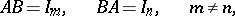where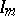and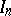denote the unit matrices of ordersand, respectively. However, non-uniqueness holds only for finite bases; if the rank of a free module is infinite, then all bases have the same cardinality. In addition, over rings that admit a homomorphism into a skew-field (in particular, over commutative rings), the rank of a free module is always uniquely defined.

A ring, considered as a left module over itself, is a free module of rank 1. Every left free module is a direct sum of free modules of rank 1. Every moduleis representable as a quotient module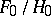of a free module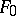. The submoduleis, in turn, representable as a quotient module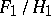of a free module. By continuing this process one obtains the exact sequencewhich is called the free resolution of. Skew-fields can be characterized as rings over which all modules are free. Over a principal ideal domain a submodule of a free module is free. Near to free modules are projective modules and flat modules (cf. Projective module; Flat module).

How to Cite This Entry:
Free module. Encyclopedia of Mathematics. URL: http://encyclopediaofmath.org/index.php?title=Free_module&oldid=13029
This article was adapted from an original article by V.E. Govorov (originator), which appeared in Encyclopedia of Mathematics - ISBN 1402006098. See original article